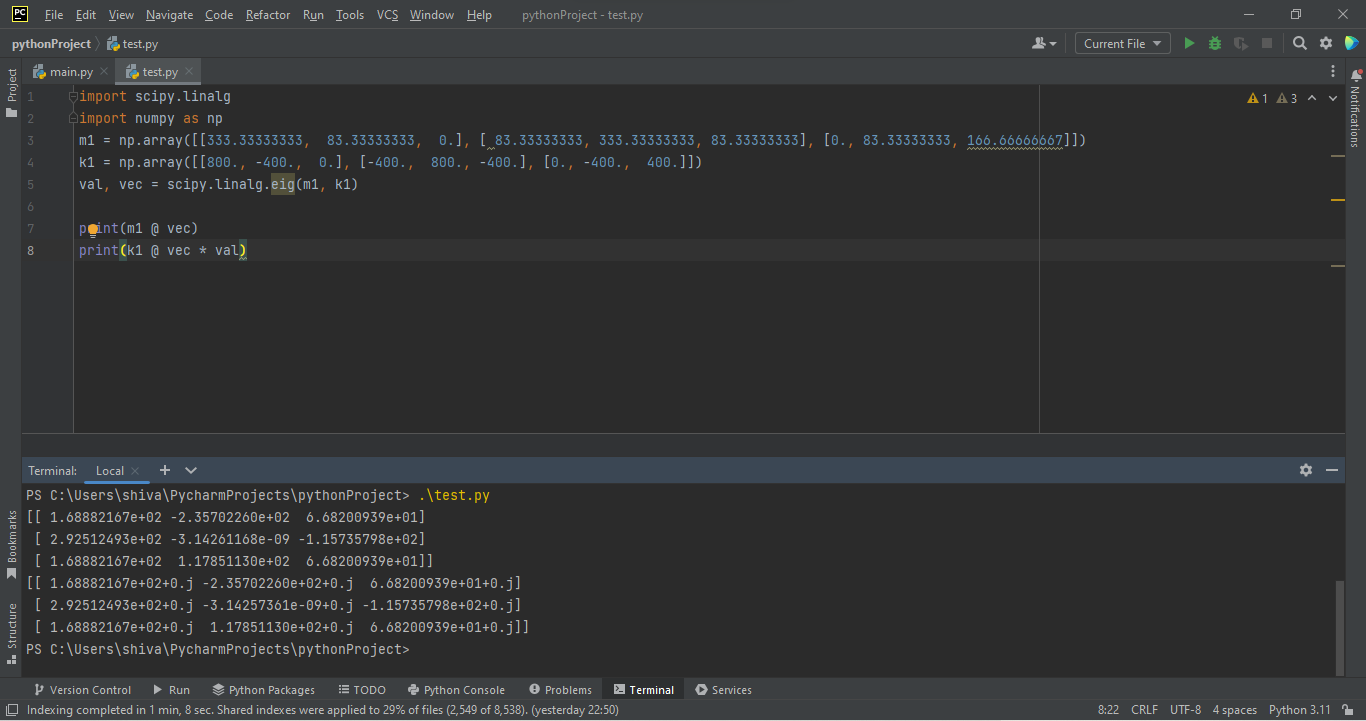# Computing the eigenvalues and eigenvectors of a matrix using SciPy's linalg.eig functionby shivanisanju03Updated: Apr 6, 2023Solution Kit

The function scipy.linalg.eig used to take a complex or a real matrix M whose eigenvalues and eigenvectors are to be evaluated. It is used to find the eigenvalues and the right or left eigenvectors of the matrix. It returns the scalar set of eigenvalues for the matrix.

Syntax: scipy.linalg.eig(a , b , left , right , overwrite_a , overwrite_b , check_finite , homogeneous_eigvals)

Please check the below code to know how to Compute the eigenvalues and eigenvectors of a matrix using SciPy's linalg.eig function.Fig: Preview of the output that you will get on running this code from your IDE

### Code

In this solution we're using numpy and scipy.linalg Library

### Instructions

Follow the steps carefully to get the output easily.

1. Install numpy,Scipy.linalg on your IDE , using the following command(Any of your favorite IDE).
2. To install numpy use ' pip install numpy '
3. To install Scipy.linalg use ' python -m pip install scipy '
4. Copy the snippet using the 'copy' and paste it in your IDE.
5. Run the file to generate the output.

NOTE: Do not copy the output given in the code snippet

I hope you found this useful. I have added the link to dependent library, version information in the following sections.

I found this code snippet by searching for ' eigen value eigen vector computations' in kandi. You can try any such use case!

### Environment tested

I tested this solution in the following versions. Be mindful of changes when working with other versions.

1. The solution is created in Pycharm 2022.3.3 (Community Edition)
2. The solution is tested on Python 3.11.1.

Using this solution, we are able to know how to Compute the eigenvalues and eigenvectors of a matrix using SciPy's linalg.eig function with simple steps. This process also facilities an easy way to use, hassle-free method to create a hands-on working version of code which would help us to know how to Compute the eigenvalues and eigenvectors of a matrix using SciPy's linalg.eig function

### Dependent Libraries

numpyby numpy

Python23755Version:v1.25.0rc1The fundamental package for scientific computing with Python.

Support
Quality
Security
Reuse

numpyby numpy

Python23755Version:v1.25.0rc1License: Permissive (BSD-3-Clause)

The fundamental package for scientific computing with Python.
Support
Quality
Security
Reuse
Jupyter Notebook0Version:CurrentSupport
Quality
Security
Reuse

scipy-linalg-exerciseby gilgamesh7

Jupyter Notebook0Version:CurrentLicense: Permissive (MIT)

Support
Quality
Security